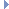﻿ MNurbsIntersector Class Reference

# MNurbsIntersector Class Reference

MNurbsIntersector Class Reference

`#include <MNurbsIntersector.h>`

## Class Description

Nurbs intersector.

The MNurbsIntersector class contains methods for efficiently finding the closest point to a NURBS surface. A tree-based algorithm is used to find the closest point.

The create() method builds the internal data required for the algorithm. As a result, calls to it should be minimized as it is a heavy operation. If the NURBS surface or matrix changes, you will need to recreate the tree based data to correspond, again using the create() method.Examples:

## Public Member Functions

MNurbsIntersector (void)
Constructor.

virtual ~MNurbsIntersector (void)
Destructor.

MStatus create (MObject &nurbsObject, const MMatrix &matrix=MMatrix::identity)
This method creates the data required by the intersector. More...

bool isCreated (void) const
This method returns true if the intersector is created. More...

MStatus getClosestPoint (const MPoint &point, MPointOnNurbs &nurbsPoint, double maxDistance=DBL_MAX) const
This method finds the closest point. More...

MStatus getIntersects (const MPoint &rayStartingPoint, const MVector &alongThisDirection, MDoubleArray &uArray, MDoubleArray &vArray, MPointArray &points, double tolerance) const
This method finds all the points of intersection of the NURBS surface with a ray (a vector at a point). More...

MStatus getIntersect (const MPoint &rayStartingPoint, const MVector &alongThisDirection, double &u, double &v, MPoint &pntOfIntersection, double tolerance) const
This method finds the first point of intersection of the NURBS surface with a ray (a vector at a point). More...

## Static Public Member Functions

static const char * className ()
Returns the name of this class. More...

## Member Function Documentation

 MStatus create ( MObject & nurbsObject, const MMatrix & matrix = `MMatrix::identity` )

This method creates the data required by the intersector.

It is a heavy operation that should be called only when necessary, such as when the underlying NURBS surface or the matrix changes.

Parameters
 [in] nurbsObject a NURBS surface object . [in] matrix specify the mapping to apply for transforming points that are specified in the getClosestPoint method into the object space of the NURBS surface.
Returns
Status code
Status Codes:Examples:
 bool isCreated ( void ) const

This method returns true if the intersector is created.

False is returned otherwise.

 MStatus getClosestPoint ( const MPoint & point, MPointOnNurbs & nurbsPoint, double maxDistance = `DBL_MAX` ) const

This method finds the closest point.

Information is returned in the nurbsPoint object.

Parameters
 [in] point Specifies the location for which to evaluate the closest point on the NURBS surface. `point' is transformed using the matrix parameter passed to the create() method, so for example if your matrix maps world to object space, then `point' should be specified in world space. [out] nurbsPoint Resulting closest point in object space. [in] maxDistance Maximum distance to consider in object space.
Returns
Status code
Status Codes:Examples:
 MStatus getIntersects ( const MPoint & rayStartingPoint, const MVector & alongThisDirection, MDoubleArray & uArray, MDoubleArray & vArray, MPointArray & points, double tolerance ) const

This method finds all the points of intersection of the NURBS surface with a ray (a vector at a point).

The points are returned in the points object, and the U and V parameters of the points are returned in the uArray and vArray objects respectively.

Parameters
 [in] rayStartingPoint Starting location of ray to test [in] alongThisDirection Direction of ray to test [in] uArray U parameters of intersection (if any) [in] vArray V parameters of intersection (if any) [out] points Storage for points of intersection (if any) [in] tolerance The epsilon value in the calculation
Returns
Status code
Status Codes:
 MStatus getIntersect ( const MPoint & rayStartingPoint, const MVector & alongThisDirection, double & u, double & v, MPoint & pntOfIntersection, double tolerance ) const

This method finds the first point of intersection of the NURBS surface with a ray (a vector at a point).

The point is returned in the pntOfIntersection object, and the U and V parameters of the point are returned in the u and v variables respectively.

Parameters
 [in] rayStartingPoint Starting location of ray to test [in] alongThisDirection Direction of ray to test [in] u U parameter of intersection (if any) [in] v V parameter of intersection (if any) [out] pntOfIntersection Storage for closest point of intersection (if any) [in] tolerance The epsilon value in the calculation
Returns
Status code
Status Codes:Examples:
 const char * className ( )
static

Returns the name of this class.

Returns
Name of this class.

The documentation for this class was generated from the following files:
• MNurbsIntersector.h
• MNurbsIntersector.cpp